Next: Electromagnetic Interaction Up: Dirac Equation Previous: Current Conservation

# Dirac Particle at Rest

We search for a solution to the Dirac equation for a particle at rest. At rest, a particle has an infinitely large de Broglie wavelength and the wave function is uniform over all space (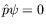). Therefore the Dirac equation reduces to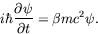(5.17)

For our representation of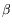(equation 5.10), the solutions are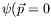):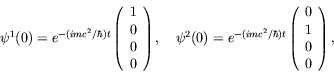(5.18)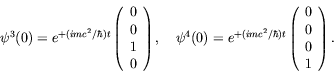(5.19)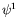and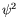are positive-energy solutions, while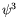and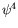are negative-energy solutions.

Douglas M. Gingrich (gingrich@ ualberta.ca)
2004-03-18